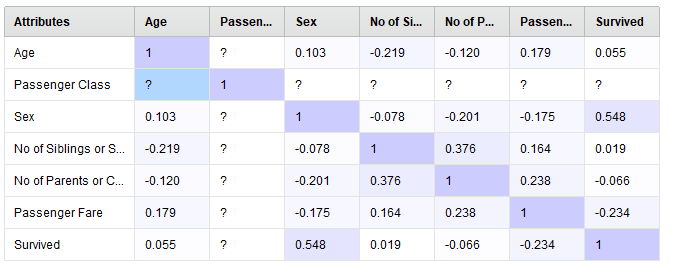# Finding correlations between outputs and inputs for large number of data

Member Posts: 1Newbie
Hi everyone!

I'm very new at Rapidminer and I have a question (I just installed it and started toying around with the "auto-model").

What I'm trying to achieve is : I did various tests by variating some inputs, and I have an excel file with for each tests the used inputs, the outputs (temperature, forces...). Since I have a large number of tests, I would like an analysis using a software like rapidminer. I would like to find correlation between inputs and outputs (like I have lower forces for this kind of tests... things like that).

I'm not quite sure if rapidminer is suitable for this? If this kind of analysis is achievable through rapidminer, I would really appreciate if you could indicate me some tutoriel to achieve this or give me some advices here (english is not my first language as you may have noticed and I have difficulties to find something that match my problem. So far on the forum I just found some posts suggesting using auto-model).

Have a good day.

Tagged:

• Moderator, Member Posts: 1,207Unicorn
Hello @maelt

If you are trying to find a correlation between attributes(including output labels), You can use Correlation matrix operator in RapidMiner which provides you with a correlation matrix. In the below scenario I selected Titanic training dataset from samples which have an output label "Survived". I included this so that I can find the correlation between inputs and output. I also provided XML code below for your understanding. You can also observe which of these are highly correlated based on their coloring.```<?xml version="1.0" encoding="UTF-8"?><process version="9.2.001">
<context>
<input/>
<output/>
<macros/>
</context>
<operator activated="true" class="process" compatibility="9.2.001" expanded="true" name="Process">
<parameter key="logverbosity" value="init"/>
<parameter key="random_seed" value="2001"/>
<parameter key="send_mail" value="never"/>
<parameter key="process_duration_for_mail" value="30"/>
<parameter key="encoding" value="SYSTEM"/>
<process expanded="true">
<operator activated="true" class="retrieve" compatibility="9.2.001" expanded="true" height="68" name="Retrieve Titanic Training" width="90" x="112" y="238">
<parameter key="repository_entry" value="//Samples/data/Titanic Training"/>
</operator>
<operator activated="true" class="concurrency:correlation_matrix" compatibility="9.2.001" expanded="true" height="103" name="Correlation Matrix" width="90" x="380" y="238">
<parameter key="attribute_filter_type" value="all"/>
<parameter key="attribute" value=""/>
<parameter key="attributes" value=""/>
<parameter key="use_except_expression" value="false"/>
<parameter key="value_type" value="attribute_value"/>
<parameter key="use_value_type_exception" value="false"/>
<parameter key="except_value_type" value="time"/>
<parameter key="block_type" value="attribute_block"/>
<parameter key="use_block_type_exception" value="false"/>
<parameter key="except_block_type" value="value_matrix_row_start"/>
<parameter key="invert_selection" value="false"/>
<parameter key="include_special_attributes" value="true"/>
<parameter key="normalize_weights" value="true"/>
<parameter key="squared_correlation" value="false"/>
</operator>
<connect from_op="Retrieve Titanic Training" from_port="output" to_op="Correlation Matrix" to_port="example set"/>
<connect from_op="Correlation Matrix" from_port="example set" to_port="result 2"/>
<connect from_op="Correlation Matrix" from_port="matrix" to_port="result 1"/>
<portSpacing port="source_input 1" spacing="0"/>
<portSpacing port="sink_result 1" spacing="0"/>
<portSpacing port="sink_result 2" spacing="0"/>
<portSpacing port="sink_result 3" spacing="0"/>
</process>
</operator>
</process>
```
Please inform if this is not what you are looking for.

Thanks

Regards,
Varun
https://www.varunmandalapu.com/